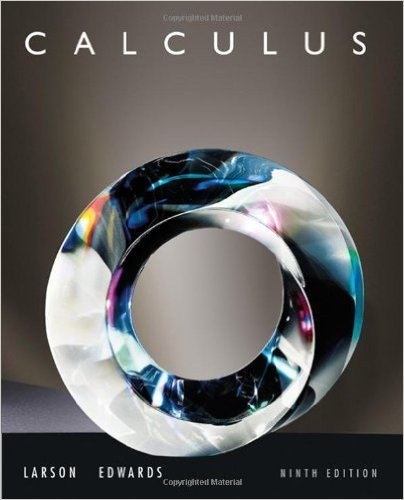×
×

# Solutions for Chapter 5.7: Inverse Trigonometric Functions: Integration## Full solutions for Calculus | 9th Edition

ISBN: 9780547167022Solutions for Chapter 5.7: Inverse Trigonometric Functions: Integration

Solutions for Chapter 5.7
4 5 0 253 Reviews
10
0
##### ISBN: 9780547167022

Since 92 problems in chapter 5.7: Inverse Trigonometric Functions: Integration have been answered, more than 63900 students have viewed full step-by-step solutions from this chapter. Chapter 5.7: Inverse Trigonometric Functions: Integration includes 92 full step-by-step solutions. Calculus was written by and is associated to the ISBN: 9780547167022. This textbook survival guide was created for the textbook: Calculus , edition: 9. This expansive textbook survival guide covers the following chapters and their solutions.

Key Calculus Terms and definitions covered in this textbook
• Absolute value of a complex number

The absolute value of the complex number z = a + b is given by ?a2+b2; also, the length of the segment from the origin to z in the complex plane.

• Anchor

See Mathematical induction.

• Common logarithm

A logarithm with base 10.

• Direction vector for a line

A vector in the direction of a line in three-dimensional space

• Higher-degree polynomial function

A polynomial function whose degree is ? 3

• Hypotenuse

Side opposite the right angle in a right triangle.

• Monomial function

A polynomial with exactly one term.

• Period

See Periodic function.

• Principal nth root

If bn = a, then b is an nth root of a. If bn = a and a and b have the same sign, b is the principal nth root of a (see Radical), p. 508.

• Projectile motion

The movement of an object that is subject only to the force of gravity

• Quotient polynomial

See Division algorithm for polynomials.

• Reduced row echelon form

A matrix in row echelon form with every column that has a leading 1 having 0’s in all other positions.

• Response variable

A variable that is affected by an explanatory variable.

• Statute mile

5280 feet.

• Sum of an infinite geometric series

Sn = a 1 - r , |r| 6 1

• Supply curve

p = ƒ(x), where x represents production and p represents price

• Tangent

The function y = tan x

• Terminal point

See Arrow.

• Terms of a sequence

The range elements of a sequence.

• Velocity

A vector that specifies the motion of an object in terms of its speed and direction.

×